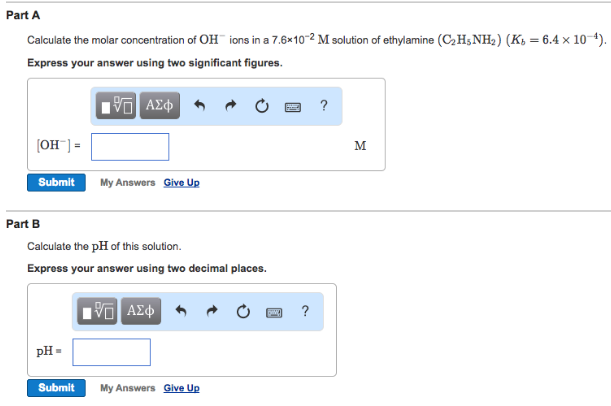# Problem: Part ACalculate the molar concentration of OH- ions in a 7.6 x 10-2 M solution of ethylamine (C2H5NH2) (Kb = 6.4 x 10-4). Express your answer using two significant figures. Part B Calculate the pH of this solution. Express your answer using two decimal places.

###### FREE Expert Solution###### Problem Details

Part A

Calculate the molar concentration of OH- ions in a 7.6 x 10-2 M solution of ethylamine (C2H5NH2) (Kb = 6.4 x 10-4). Express your answer using two significant figures.

Part B

Calculate the pH of this solution.